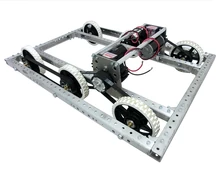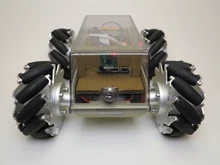## FANDOM

47 Pages

There are several different wheel configurations for driving. Each needs to be set up differently and programmed in a unique manner. In the following, we assume two joysticks called left and right. We also assume the four wheels fl, fr, bl, br (f = front, b = back, l = left, r = right). Note that much of the code depends on wiring, so you should write it yourself from scratch (basically, I just assume counter-clockwise is positive).

## StandardThis is the generic layout consisting of two parallel sets of wheels as shown.

### Arcade

Arcade drive is what most drivers would consider intuitive. The left joystick generally controls forward speed; while the right joystick controls rotational velocity.

```public void arcadeDrive(Joystick left, Joystick right) {
// max makes sure neither of the speeds exceed 1.0
double left_speed = left.getY() + right.getX(),
right_speed = -left.getY() + right.getX(),
max = Math.max(Math.abs(left_speed), Math.abs(right_speed));
left_speed /= Math.max(max, 1);
right_speed /= Math.max(max, 1);

fl_motor.set(left_speed);
bl_motor.set(left_speed);
fr_motor.set(right_speed);
br_motor.set(right_speed);
}```

### Tank

Tank is the easiest configuration to program, since it sends the left joystick's value to the left wheels and the right joystick's values to the right wheels.

```public void tankDrive(Joystick left, Joystick right) {
fl_motor.set(left.getY());
bl_motor.set(left.getY());
fr_motor.set(-right.getY()); //Negative to correct direction
br_motor.set(-right.getY());
}```

## MecanumMecanum wheels have small wheels on main wheels, which allow for complete control of both rotation and translation.

//Todo finish this